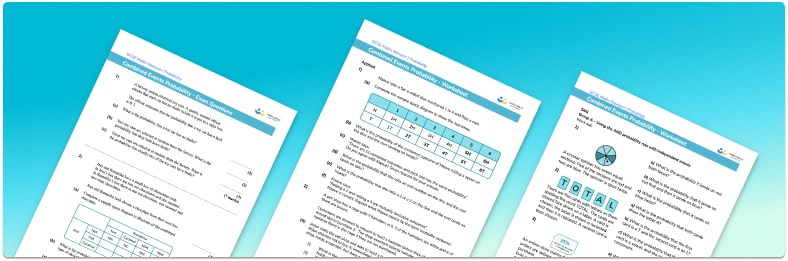# Probability Of Events Worksheet• Section 1 of the probability of events worksheet contains 20+ skills-based probability of events questions, in 3 groups to support differentiation
• Section 2 contains 4 applied probability of events questions with a mix of worded problems and deeper problem solving questions
• Section 3 contains 3 foundation and higher level GCSE exam style probability of events questions
• Answers and a mark scheme for all probability of events questions are provided
• Questions follow variation theory with plenty of opportunities for students to work independently at their own level
• All questions created by fully qualified expert secondary maths teachers

Suitable for GCSE maths revision for AQA, OCR and Edexcel exam boards

• This field is for validation purposes and should be left unchanged.

You can unsubscribe at any time (each email we send will contain an easy way to unsubscribe). To find out more about how we use your data, see our privacy policy.

### Probability of events at a glance

In probability, combined events are two or more events that occur together, for example picking a King from a deck of cards and rolling an even number on a fair dice. For basic probability of a single event, the probability of an event occurring is equal to the number of desired outcomes divided by the total number of possible outcomes. When working with combined events, we must be aware that the outcome of one event may change the probability of another event.

When calculating probabilities for combined events we need to use the following rules:

For mutually exclusive events, P(A or B) = P(A) + P(B).

For independent events, P(A and B) = P(A) ✖ P(B).

We often represent combined events using a tree diagram to make the different possibilities easier to visualise.

Consider a spinner where the probability of landing on blue is 3 out of 5 and the probability of landing on green is 2 out of 5. We could find the probability of landing on the same colour twice. The probability of landing on blue twice is P(blue and blue) = 0.6 ✖ 0.6 = 0.36. The probability of landing on green twice, P(green and green) = 0.4 ✖ 0.4 = 0.16. The total probability of landing on the same colour twice is 0.36 + 0.16 = 0.52.

Another example is the probability of choosing 2 red balls from a bag containing 3 white balls and 7 red balls. The probability of event A, picking 1 red ball is 7 out of 10. Once we have picked one red ball, the probability of event B, picking a second red ball, is 6 out of 9, since there are now 6 red balls and 3 white balls remaining. The total probability of picking 2 red balls is the product of these two values: 7 out of 10, multiplied by 6 out of 9, is equal to 42 out of 90.

It is useful to know different types of numbers such as odd numbers, even numbers and prime numbers as you may need to use these facts when calculating probabilities of outcomes when rolling a die, picking a card from a pack of playing cards, or by simply selecting a type of number from a list. These can all be used with combined events.

Looking forward, students can then progress to additional probability worksheets, for example the conditional probability worksheet, the mutually exclusive events worksheet, or the experimental probability worksheet.For more teaching and learning support on probability our GCSE maths lessons provide step by step support for all GCSE maths concepts.

## Do you have KS4 students who need more focused attention to succeed at GCSE?There will be students in your class who require individual attention to help them succeed in their maths GCSEs. In a class of 30, it’s not always easy to provide.

Help your students feel confident with exam-style questions and the strategies they’ll need to answer them correctly with our dedicated GCSE maths revision programme.

Lessons are selected to provide support where each student needs it most, and specially-trained GCSE maths tutors adapt the pitch and pace of each lesson. This ensures a personalised revision programme that raises grades and boosts confidence.

Find out more